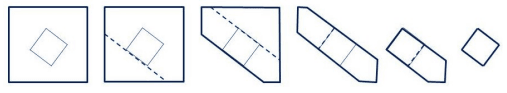### A - Cut It Out!

##### Languages: C, C++, Java, Python, ... (details)

You are given two convex polygons $A$ and $B$. It is guaranteed that $B$ is strictly contained inside of $A$.

You would like to make a sequence of cuts to cut out $B$ from $A$. To do this, you draw a straight line completely through $A$ that is incident to one of the edges of $B$, which separates $A$ into two pieces. You cut along this line and discard the piece that doesn’t contain $B$. You repeat this until the piece that you have left is exactly $B$.The cost of making a cut is equal to the length of the cut (i.e. the length of the line through the remainder of $A$). Given $A$ and $B$, find the minimum cost needed to cut $B$ out.

#### Input

Each input will consist of a single test case. Note that your program may be run multiple times on different inputs. Each test case will begin with a line containing a single integer $a$ $(3 \leq a \leq 200)$, which is the number of points in polygon $A$. Each of the next a lines will contain two integers $x$ and $y$ $(-10^6 \leq x, y \leq 10^6)$, which are the vertices of polygon $A$, in clockwise order. It is guaranteed that polygon $A$ will be convex.

The next line will contain a single integer $b$ $(3 \leq b \leq 200)$, which is the number of points in polygon $B$. Each of the next $b$ lines will contain two integers $x$ and $y$ $(-10^6 \lt x, y \lt 10^6)$, which are the vertices of polygon $B$, in clockwise order. It is guaranteed that polygon $B$ will be convex. It is also guaranteed that polygon $B$ will reside entirely within the interior of polygon $A$. No three points, within a polygon or across polygons, will be collinear.

#### Output

Output a single floating point number, which is the minimum cost to cut $B$ out of $A$. To be considered correct, this number must be within a relative error of $10^{−6}$ of the judges’ answer.

#### Sample test(s)

Input
4 0 0 0 14 15 14 15 0 4 8 3 4 6 7 10 11 7
Output
40.0000000000
Input
4 -100 -100 -100 100 100 100 100 -100 8 -1 -2 -2 -1 -2 1 -1 2 1 2 2 1 2 -1 1 -2
Output
322.1421356237